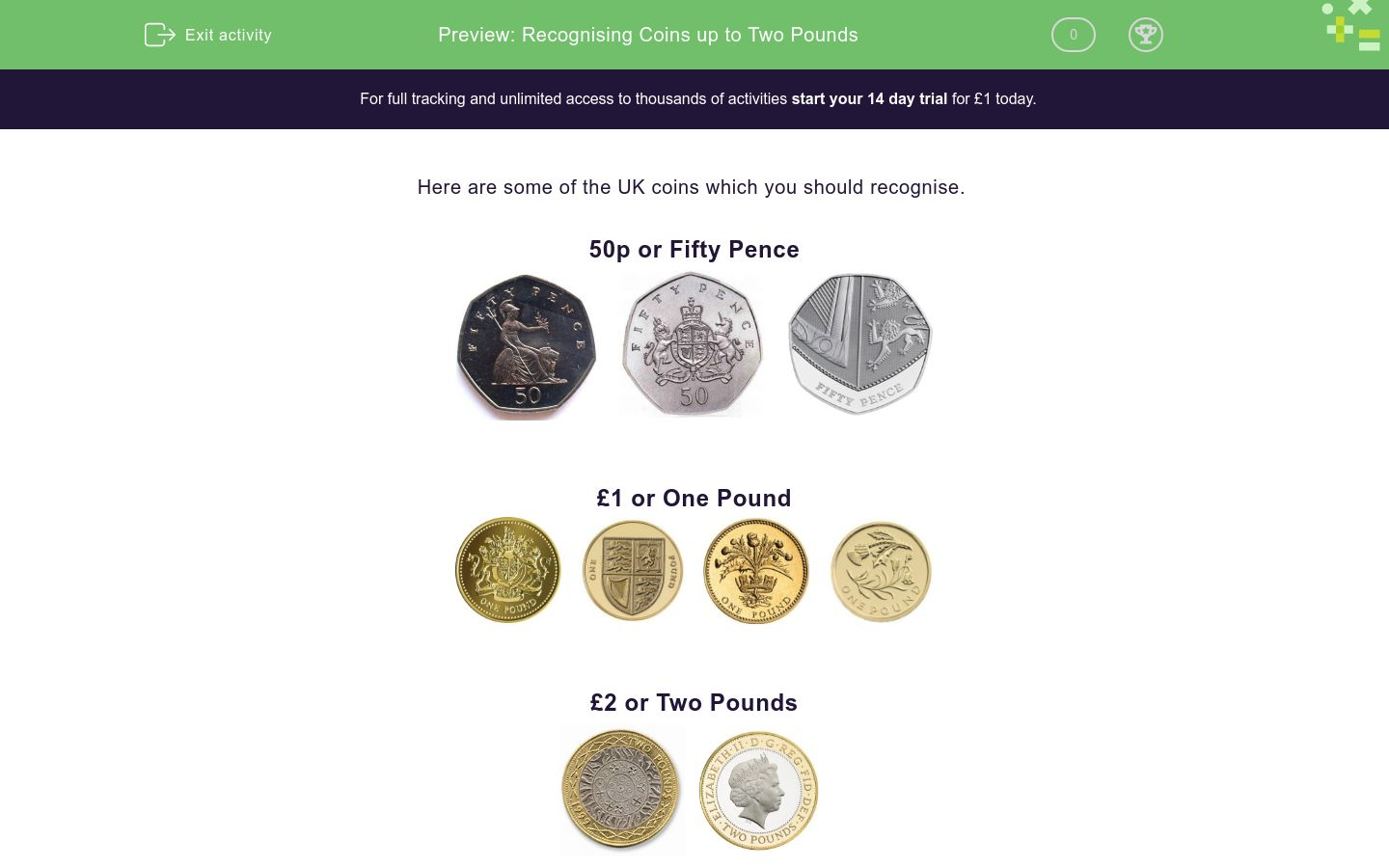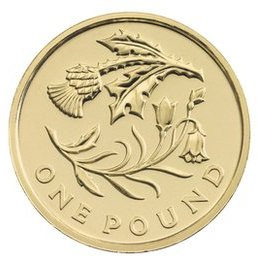# Recognising Coins up to Two Pounds

In this worksheet, students must recognise 50 pence, 1 and 2 pound coins.Key stage:  KS 1

Curriculum topic:   Measurement

Curriculum subtopic:   Money and its Value

Difficulty level:### QUESTION 1 of 10

Here are some of the UK coins which you should recognise.

50p or Fifty Pence£1 or One Pound£2 or Two PoundsHow much is this coin worth?1p

2p

5p

50p

£1

How much is this coin worth?1p

£1

5p

2p

£2

How much is this coin worth?£1

£2

5p

2p

1p

How much is this coin worth?20p

10p

5p

£1

1p

How much is this coin worth?20p

10p

5p

50p

1p

How much is this coin worth?50p

10p

5p

£2

£1

How much is this coin worth?50p

£5

£2

2p

£1

How much is this coin worth?£2

10p

50p

£1

1p

How much is this coin worth?50p

£2

5p

£5

£1

How much is this coin worth?£5

£2

£1

50p

1p

• Question 1

How much is this coin worth?50p
EDDIE SAYS
This is a Fifty Pence coin.
• Question 2

How much is this coin worth?£2
EDDIE SAYS
This is a Two Pound coin.
• Question 3

How much is this coin worth?£1
EDDIE SAYS
This is a One Pound coin.
• Question 4

How much is this coin worth?£1
EDDIE SAYS
This is a One Pound coin.
• Question 5

How much is this coin worth?50p
EDDIE SAYS
This is a Fifty Pence coin.
• Question 6

How much is this coin worth?£1
EDDIE SAYS
This is a One Pound coin.
• Question 7

How much is this coin worth?£2
EDDIE SAYS
This is a Two Pound coin.
• Question 8

How much is this coin worth?£1
EDDIE SAYS
This is a One Pound coin.
• Question 9

How much is this coin worth?50p
EDDIE SAYS
This is a Fifty Pence coin.
• Question 10

How much is this coin worth?£1
EDDIE SAYS
This is a One Pound coin.
---- OR ----

Sign up for a £1 trial so you can track and measure your child's progress on this activity.

### What is EdPlace?

We're your National Curriculum aligned online education content provider helping each child succeed in English, maths and science from year 1 to GCSE. With an EdPlace account you’ll be able to track and measure progress, helping each child achieve their best. We build confidence and attainment by personalising each child’s learning at a level that suits them.

Get started